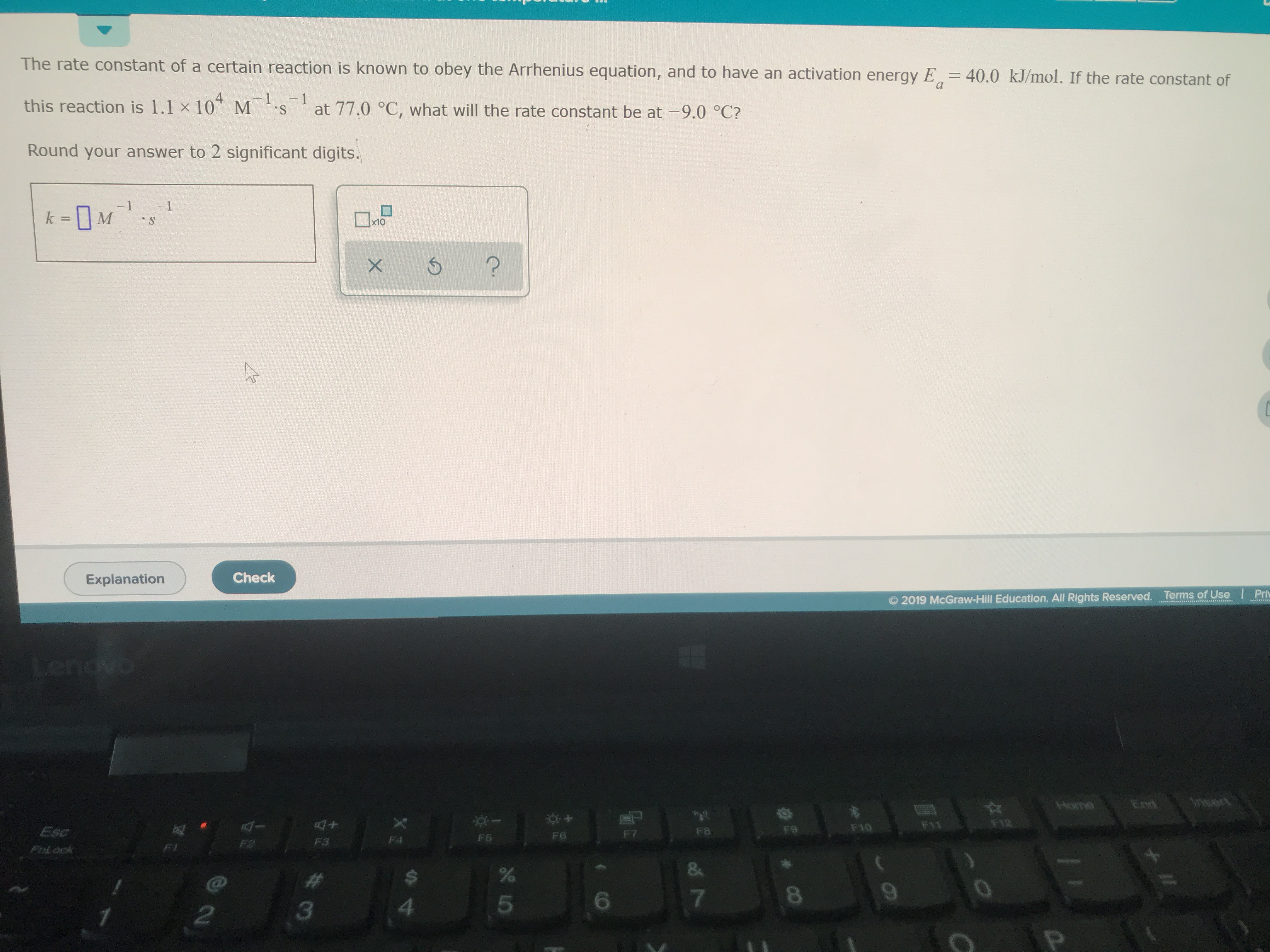# The rate constant of a certain reaction is known to obey the Arrhenius equation, and to have an activation energy Ea = 40.0 kJ/mol. If the rate constant of this reaction is 1.1x10^4 M^-1 s^-1 at 77.0 C, what will be the rate constant at -9.0 C? Round your answer to 2 significant digits.